### Home > A2C > Chapter 7 > Lesson 7.1.5 > Problem7-85

7-85.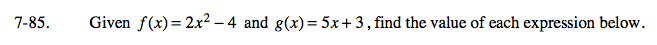Substitute the quantity inside the parentheses ( ) for x into the f(x) or g(x) equation and simplify.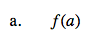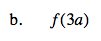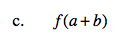f(a + b) = 2a2 + 4ab + 2b2 − 4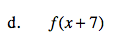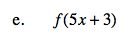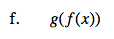f(g(x)) = 10x2 − 17